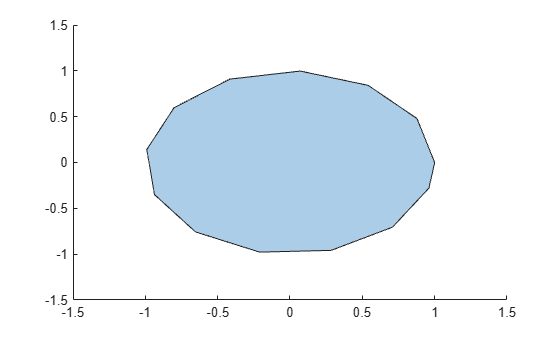# numsides

Number of `polyshape` sides

## Syntax

``N = numsides(polyin)``
``N = numsides(polyin,I)``

## Description

example

````N = numsides(polyin)` returns the number of sides of a `polyshape` object.```
````N = numsides(polyin,I)` returns the number of sides of the `I`th boundary of `polyin`.This syntax is only supported when `polyin` is a scalar `polyshape` object.```

## Examples

collapse all

Create a polygon and determine its number of sides.

```t = 0:0.5:2*pi; P = [cos(t)' sin(t)']; polyin = polyshape(P); plot(polyin)````N = numsides(polyin)`
```N = 13 ```

## Input Arguments

collapse all

Input `polyshape`, specified as a scalar, vector, matrix, or multidimensional array.

Data Types: `polyshape`

Boundary index, specified as a scalar integer or vector of integers. Each element of `I` corresponds to a single boundary of the input `polyshape`.

Data Types: `double` | `single` | `int8` | `int16` | `int32` | `int64` | `uint8` | `uint16` | `uint32` | `uint64`